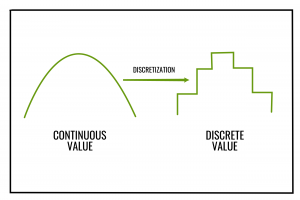Related Articles

# ML | Binning or Discretization

• Last Updated : 13 May, 2019

Real-world data tend to be noisy. Noisy data is data with a large amount of additional meaningless information in it called noise. Data cleaning (or data cleansing) routines attempt to smooth out noise while identifying outliers in the data.

There are three data smoothing techniques as follows –

1. Binning : Binning methods smooth a sorted data value by consulting its “neighborhood”, that is, the values around it.
2. Regression : It conforms data values to a function. Linear regression involves finding the “best” line to fit two attributes (or variables) so that one attribute can be used to predict the other.
3. Outlier analysis : Outliers may be detected by clustering, for example, where similar values are organized into groups, or “clusters”. Intuitively, values that fall outside of the set of clusters may be considered as outliers.

Binning method for data smoothing –
Here, we are concerned with the Binning method for data smoothing. In this method the data is first sorted and then the sorted values are distributed into a number of buckets or bins. As binning methods consult the neighborhood of values, they perform local smoothing.

There are basically two types of binning approaches –

1. Equal width (or distance) binning : The simplest binning approach is to partition the range of the variable into k equal-width intervals. The interval width is simply the range [A, B] of the variable divided by k,
`w = (B-A) / k`

Thus, ith interval range will be `[A + (i-1)w, A + iw]` where i = 1, 2, 3…..k
Skewed data cannot be handled well by this method.

2. Equal depth (or frequency) binning : In equal-frequency binning we divide the range [A, B] of the variable into intervals that contain (approximately) equal number of points; equal frequency may not be possible due to repeated values.

#### How to perform smoothing on the data?

There are three approaches to perform smoothing –

1. Smoothing by bin means : In smoothing by bin means, each value in a bin is replaced by the mean value of the bin.
2. Smoothing by bin median : In this method each bin value is replaced by its bin median value.
3. Smoothing by bin boundary : In smoothing by bin boundaries, the minimum and maximum values in a given bin are identified as the bin boundaries. Each bin value is then replaced by the closest boundary value.

Sorted data for price(in dollar) : 2, 6, 7, 9, 13, 20, 21, 25, 30

```Partition using equal frequency approach:
Bin 1 : 2, 6, 7
Bin 2 : 9, 13, 20
Bin 3 : 21, 24, 30

Smoothing by bin mean :
Bin 1 : 5, 5, 5
Bin 2 : 14, 14, 14
Bin 3 : 25, 25, 25

Smoothing by bin median :
Bin 1 : 6, 6, 6
Bin 2 : 13, 13, 13
Bin 3 : 24, 24, 24

Smoothing by bin boundary :
Bin 1 : 2, 7, 7
Bin 2 : 9, 9, 20
Bin 3 : 21, 21, 30
```

Binning can also be used as a discretization technique. Here discretization refers to the process of converting or partitioning continuous attributes, features or variables to discretized or nominal attributes/features/variables/intervals.
For example, attribute values can be discretized by applying equal-width or equal-frequency binning, and then replacing each bin value by the bin mean or median, as in smoothing by bin means or smoothing by bin medians, respectively. Then the continuous values can be converted to a nominal or discretized value which is same as the value of their corresponding bin.Below is the Python implementation:

## bin_mean

 `import` `numpy as np``from` `sklearn.linear_model ``import` `LinearRegression``from` `sklearn ``import` `linear_model``# import statsmodels.api as sm``import` `statistics``import` `math``from` `collections ``import` `OrderedDict`` ` `x ``=``[]``print``(``"enter the data"``)``x ``=` `list``(``map``(``float``, ``input``().split()))`` ` `print``(``"enter the number of bins"``)``bi ``=` `int``(``input``())`` ` `# X_dict will store the data in sorted order``X_dict ``=` `OrderedDict()``# x_old will store the original data``x_old ``=``{}``# x_new will store the data after binning``x_new ``=``{}`` ` ` ` `for` `i ``in` `range``(``len``(x)):``    ``X_dict[i]``=` `x[i]``    ``x_old[i]``=` `x[i]`` ` `x_dict ``=` `sorted``(X_dict.items(), key ``=` `lambda` `x: x[``1``])`` ` `# list of lists(bins)``binn ``=``[]``# a variable to find the mean of each bin``avrg ``=` `0`` ` `i ``=` `0``k ``=` `0``num_of_data_in_each_bin ``=` `int``(math.ceil(``len``(x)``/``bi))`` ` `# performing binning``for` `g, h ``in` `X_dict.items():``    ``if``(i

## bin_median

 `import` `numpy as np``from` `sklearn.linear_model ``import` `LinearRegression``from` `sklearn ``import` `linear_model``# import statsmodels.api as sm``import` `statistics``import` `math``from` `collections ``import` `OrderedDict`` ` ` ` `x ``=``[]``print``(``"enter the data"``)``x ``=` `list``(``map``(``float``, ``input``().split()))`` ` `print``(``"enter the number of bins"``)``bi ``=` `int``(``input``())`` ` `# X_dict will store the data in sorted order``X_dict ``=` `OrderedDict()``# x_old will store the original data``x_old ``=``{}``# x_new will store the data after binning``x_new ``=``{}`` ` `for` `i ``in` `range``(``len``(x)):``    ``X_dict[i]``=` `x[i]``    ``x_old[i]``=` `x[i]`` ` `x_dict ``=` `sorted``(X_dict.items(), key ``=` `lambda` `x: x[``1``])`` ` ` ` `# list of lists(bins)``binn ``=``[]``# a variable to find the mean of each bin``avrg ``=``[]`` ` `i ``=` `0``k ``=` `0``num_of_data_in_each_bin ``=` `int``(math.ceil(``len``(x)``/``bi))``# performing binning``for` `g, h ``in` `X_dict.items():``    ``if``(i

## bin_boundary

 `import` `numpy as np``from` `sklearn.linear_model ``import` `LinearRegression``from` `sklearn ``import` `linear_model``# import statsmodels.api as sm``import` `statistics``import` `math``from` `collections ``import` `OrderedDict`` ` `x ``=``[]``print``(``"enter the data"``)``x ``=` `list``(``map``(``float``, ``input``().split()))`` ` `print``(``"enter the number of bins"``)``bi ``=` `int``(``input``())`` ` `# X_dict will store the data in sorted order``X_dict ``=` `OrderedDict()``# x_old will store the original data``x_old ``=``{}``# x_new will store the data after binning``x_new ``=``{}`` ` ` ` `for` `i ``in` `range``(``len``(x)):``    ``X_dict[i]``=` `x[i]``    ``x_old[i]``=` `x[i]`` ` `x_dict ``=` `sorted``(X_dict.items(), key ``=` `lambda` `x: x[``1``])`` ` `# list of lists(bins)``binn ``=``[]``# a variable to find the mean of each bin``avrg ``=``[]`` ` `i ``=` `0``k ``=` `0``num_of_data_in_each_bin ``=` `int``(math.ceil(``len``(x)``/``bi))`` ` `for` `g, h ``in` `X_dict.items():``    ``if``(i``=` `abs``(h``-``binn[j][``1``])):``            ``x_new[g]``=` `binn[j][``1``]``            ``i ``=` `i ``+` `1``        ``else``:``            ``x_new[g]``=` `binn[j][``0``]``            ``i ``=` `i ``+` `1``    ``else``:``        ``i ``=` `0``        ``j ``=` `j ``+` `1``        ``if``(``abs``(h``-``binn[j][``0``]) >``=` `abs``(h``-``binn[j][``1``])):``            ``x_new[g]``=` `binn[j][``1``]``        ``else``:``            ``x_new[g]``=` `binn[j][``0``]``        ``i ``=` `i ``+` `1`` ` `print``(``"number of data in each bin"``)``print``(math.ceil(``len``(x)``/``bi))``for` `i ``in` `range``(``0``, ``len``(x)):``    ``print``(``'index {2} old value  {0} new value  {1}'``.``format``(x_old[i], x_new[i], i))`

Reference: https://en.wikipedia.org/wiki/Data_binning

Attention reader! Don’t stop learning now. Get hold of all the important Machine Learning Concepts with the Machine Learning Foundation Course at a student-friendly price and become industry ready.

My Personal Notes arrow_drop_up